# Operating principle of the LISST-25 constant calibration sediment sensor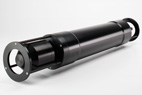LISST-25X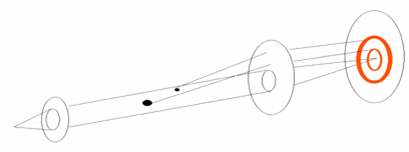Figure 1. From left to right, a laser, collimating lens, scattering particles, receiving lens and the scattered light on a detector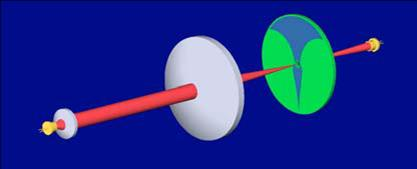Figure 2. The laser, lenses and the detector. The lower half of the detector is comet shaped.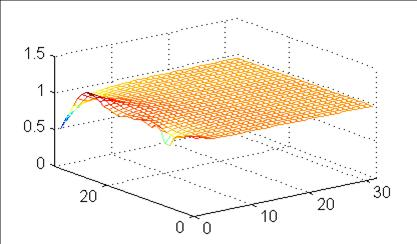Figure 3. The ratio of estimated to true concentration against mean
size (left abscissa) and size spread (right abscissa) of simulated distributions.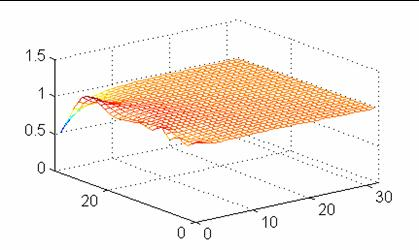Figure 4. The ratio of estimated to true Sauter Mean Diameter
against mean size (left abscissa) and size spread (right abscissa) of simulated distributions.

Operating principle of the LISST-25 constant calibration sediment sensor
[Sequoia, May 1, 2008; based an Application Note L005]
 The LISST-25 instrument is based on a new concept developed at Sequoia Scientific, Inc. (patent pending) that exploits the multi-angle scattering properties of particles. This instrument marks a departure from the simplistic light-source-detector technology employed in previous optical sediment sensors. Previous sediment sensors have employed either optical transmission or optical scattering as a surrogate for sediment concentration, ignoring the angular scattering properties of particles. Therefore, the prior sensors have produced an approximate measurement of the total particle area, not volume. The result is a non-constant calibration. The LISST-25 sums the forward-scattered light at multiple angles in a weighted manner. The weight factors are derived using Mie theory. This makes the LISST-25 a precise instrument designed to measure total suspended particle volume concentration and total particle area concentration. From the ratio of total volume and total area of particles the Sauter Mean Diameter is computed. The LISST-25 is based on two fundamental properties of light scattering by particles. First, most of the optical energy1 scattered by particles in the forward direction appears at small angles for larger particles, and vice versa for smaller particles. Scattering angle corresponds to distance from the center of the detector (Figure 1). Therefore, most of the scattered energy would appear in an annulus of a small radius for larger particles. Conversely, for smaller particles most of the scattered energy would appear in an annulus at a larger radius. This inverse relationship between particle size and the radius (or angle) at which most of the scattered energy appears is a fundamental characteristic of light scattering by particles. It permits separating the scattering from large vs. small particles based on the radius where the light appears. This is the property that is employed for particle sizing in all our LISST instruments. The second property behind the design of the LISST-25 relates to the magnitude of light scattering for a given volume of particles. Larger particles not only scatter intosmall angles as described above, they also produce less scattered energy per-unit-particle-volume than smaller particles. As an example, a single particle of size 100 microns would scatter far less light at its characteristic small angle than 8 particles of size 50 microns would at their characteristic twice larger angle although they have an equal combined volume. Any sediment sensor should produce an equal photocurrent for equal volumes of particles regardless of size. The LISST-25 achieves this by use of shaped detectors. Since smaller particles produce disproportionately large amount of light at large radii, the detector is shaped so that light is sensed over a smaller fraction of the full annulus at the larger radius. This idea leads to a decreasing angular width of the photo-active annulus away from the center. This is the origin of the comet shaped particle volume sensor (Figure 2). Total particle area concentration is sensed in a similar way, but on a separate detector. As particles of all sizes produce nearly the same amount of light per-unit-particlearea in an annulus at their corresponding radius (scattering angle) on the detector, there is only a small variation in the width of the annulus at different radii. This produces the nearly constant arc-width, wedge shaped detector for total area sensing. This is seen as the upper detector in Figure 2. Note that the hole in the center of the detector passes the focused laser beam through unobstructed. A separate, conventional photodiode senses the power in this beam. The optical transmission is estimated from the ratio of the magnitude of transmitted laser power in the presence of particles with its value in clear water. The accuracy of the estimate of sediment concentration and the estimate of the mean diameter is of fundamental interest. This is performed at two levels. In theoretical simulations it is possible to synthesize the angular scattering on the face of the detector. This simulation is done with assumed Gaussian particle size distributions. The mean and standard deviation of the simulated size-distribution isvaried from the narrowest to the broadest, in equal phisize increments. The LISST-25 measures particles in the size range of 1.25-250 microns2. The simulation is implemented by dividing this size range into 32 equal phi-size bins. From the simulated scattering on the face of the detector, the response of the special detectors is computed for the estimated mean size and the estimated concentration. These parameters are normalized to the corresponding simulated input values, so that ideally these ratios should be equal to 1. In Figure 3, we display a simulated response that is close to 1, except for the very narrow distribution of the largest sizes where it can reach a factor of 0.5. Contrasting this to prior sensors, which would suffer an error of a factor of 200, a factor of 0.5 for the LISST-25 is a dramatic improvement and the essential advantage of this instrument For natural particles, a relevant question is the effect of composition and shape on this measurement. Fortunately, composition is not a big issue with smallanglescattering – it is dominated by diffraction. Only when particles match the index of the medium (water) closely, the errors can be significant. The word shape, onthe other hand, encompasses the full diversity of shapes in nature. For this reason, it is only possible to address shape in general terms. As small angle scattering isdominated by light diffracting around particles, it implies that the scattering process is sensitive to particle crosssection. Since all shapes other than spheres have a larger surface to volume ratio than spheres, non-spherical particle concentrations will in general be overestimated.This effect is common to all optical sensors, including those based on photography. In general, shape changes or an unknown mass density may affect concentrationestimates by factors of order 1. The effect of size in contrast, can be much greater. The LISST-25 instrument overcomes this most significant error which arises from change in size of sediment particles. # # # 1In particle sizing, the term energy is used to mean optical intensity (watt/m2) integrated over area, in contrast with the conventional meaning of energy being the time-integrated power.2An optional version of the instrument covers the larger size range 2.5-500 microns.